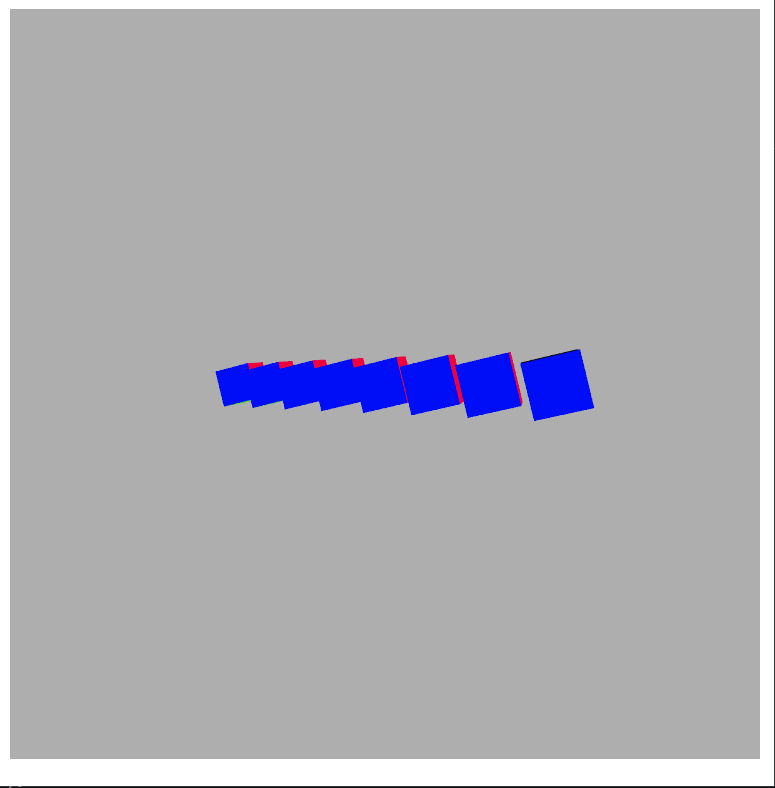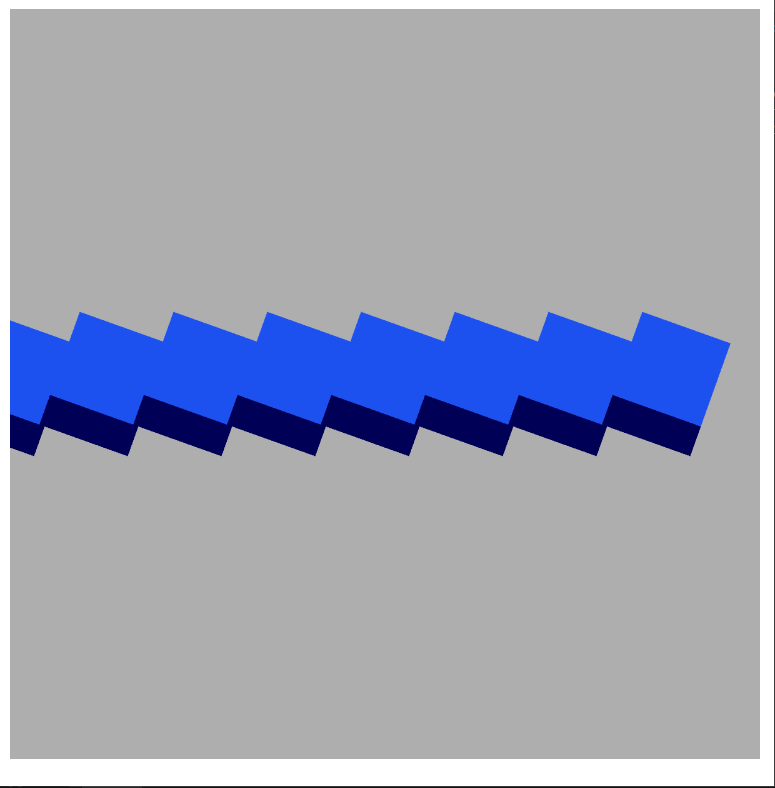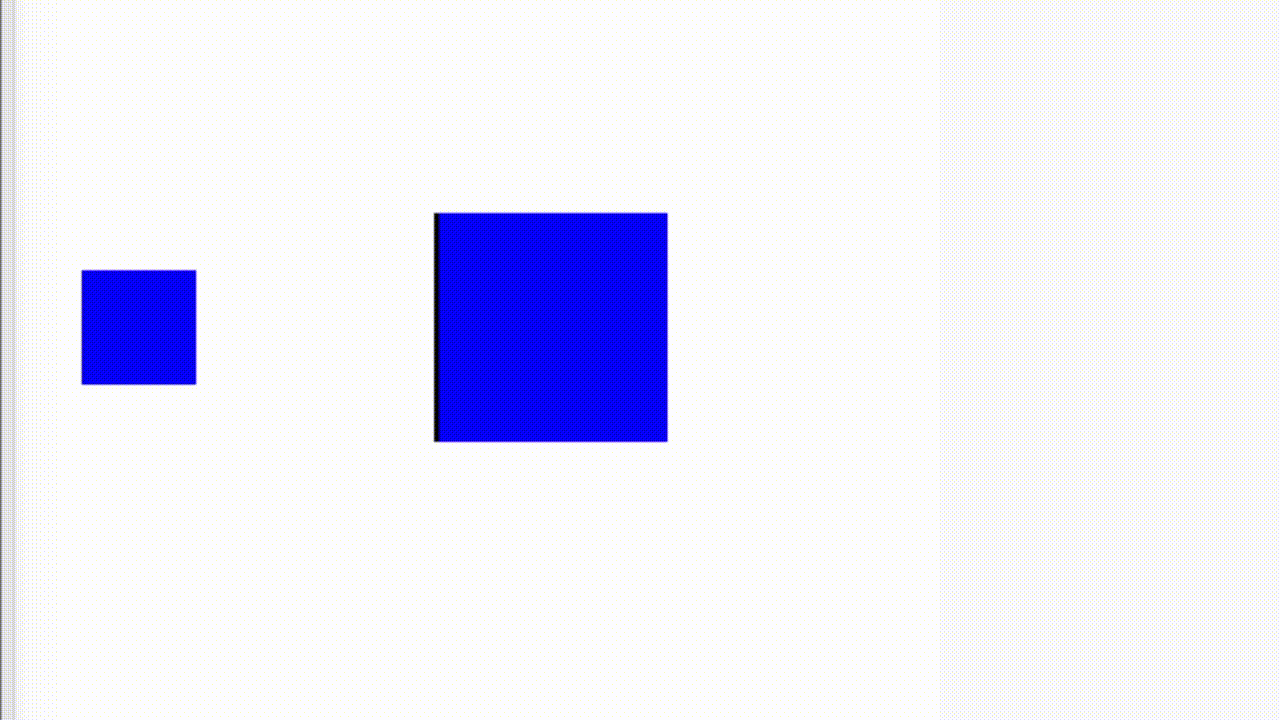# p5.js ortho() Function

• Difficulty Level : Medium
• Last Updated : 23 May, 2021

The ortho() function in p5.js is used to set the orthographic projection of a 3D sketch. In this projection, all the objects with the same dimension appear the same size, regardless of their distance from the camera. The p5.ortho() method comes under the camera section in p5.js library. The optional parameters can be used to specify the frustum of the camera in all the planes.

Syntax:

Hey geek! The constant emerging technologies in the world of web development always keeps the excitement for this subject through the roof. But before you tackle the big projects, we suggest you start by learning the basics. Kickstart your web development journey by learning JS concepts with our JavaScript Course. Now at it's lowest price ever!

`ortho([left], [right], [bottom], [top], [near], [far])`

Parameters: This function accepts six parameters as mentioned above and described below:

• left: It is a number that defines the camera’s frustum left plane. This is an optional parameter.
• right: It is a number that defines the camera’s frustum right plane. This is an optional parameter.
• bottom: It is a number that defines the camera’s frustum bottom plane. This is an optional parameter.
• top: It is a number that defines the camera’s frustum top plane.  This is an optional parameter.
• near: It is a number that defines the camera’s frustum near the plane. This is an optional parameter.
• far: It is a number that defines the camera’s frustum far plane. This is an optional parameter.

Note: Here, parameters surrounded by squared brackets are optional. And if no parameters were given then the default parameters used in ortho() are :

`ortho(-width/2, width/2, -height/2, height/2)`

The below examples illustrate the ortho() function in p5.js:

Example 1: Without using orthographic projection.

Code:

## Javascript

 `function` `setup() {``  ``// Set the canvas``  ``createCanvas(600, 600, WEBGL);``}` `function` `draw() {``  ``// Set the background``  ``background(175);` `  ``// Set the point light of the sketch``  ``pointLight(255, 255, 255, 0, -200, 200);` `  ``// Create multiple cubes in the plane``  ``for` `(let x = -200; x < 200; x += 50) {``    ``push();``    ``translate(x, 0, x - 200);` `    ``rotateZ(frameCount * 0.02);``    ``rotateX(frameCount * 0.02);``    ``normalMaterial();``    ``box(50);``    ``pop();``  ``}``}`

Output:Without using orthographic projection

Example 2: Using an orthographic projection with the ortho() function.

Code:

## Javascript

 `function` `setup() {` `  ``// Set the canvas``  ``createCanvas(600, 600, WEBGL);``}` `function` `draw() {` `  ``// Set the background``  ``background(175);` `  ``// Use the orthographic projection``  ``ortho(-200, 200, 200, -200, 0.1, 1000);``  ``pointLight(255, 255, 255, 0, -200, 200);` `  ``// Create multiple cubes in the plane``  ``for` `(let x = -200; x < 200; x += 50) {``    ``push();``    ``translate(x, 0, x - 200);` `    ``rotateZ(frameCount * 0.02);``    ``rotateX(frameCount * 0.02);``    ``normalMaterial();``    ``box(50);``    ``pop();``  ``}``}`

Output:Using Orthographic Projection

Example 3:

Code:

## Javascript

 `function` `setup() {``  ` `  ``// creating canvas with given dimensions``  ``createCanvas(400, 400,WEBGL);``  ` `  ``// calling ortho method with default parameters``  ``ortho();``}` `function` `draw() {``  ``// creating a window with white background``  ``background(255);``  ` `  ``// used to control the objects in projection``  ``orbitControl();``  ` `  ``// it will the fill the object with colors,``  ``// so we can easily distinguish between two objects``  ``normalMaterial();``  ` `  ``// push will save the current drawing``  ``push();``  ` `  ``// translate will transform``  ``// the object by given width and height``  ``translate(30, 0);``  ` `  ``// box will draw box on the screen``  ``// with width,height and depth of 100``  ``box(100);``  ` `  ``// pop will load the saved drawing``  ``pop();``  ` `  ``// push will save the current drawing``  ``push();``  ` `  ``// translate will transform``  ``// the object by given width and height``  ``translate(-150,0);``  ` `  ``// box will draw box on the screen``  ``// with width,height and depth of 100``  ``box(50);``  ` `  ``// pop will load the saved drawing``  ``pop();``}`

Output:Using Orthographic Projection

Reference: https://p5js.org/reference/#/p5/ortho

My Personal Notes arrow_drop_up